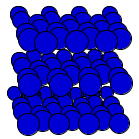Crystal Lattice Structures: Creation Date: 20 Apr 2002 Last Modified: 21 Oct 2004

# The Rhombohedral Graphite Crystal StructureYou can now

• This is a part of a revised version of the original graphite structure phase. We have preserved the old graphite structure page here.
• Graphite also comes in a hexagonal form, either flat (A9) or buckled
• Note that this structure has the same symmetry and Wyckoff positions as the arsenic (A7) structure (though we have shown that in a different orientation) and βO2.
• When z = 1/6 (shown in the figures above) we have smooth graphite sheets. Other values of z lead to buckling
• When θ = 60 degrees and z = 1/8 we get the diamond (A4) structure.
• The rhombohedral lattice can be specified by the length of the primitive vectors, a, and the angle between them, θ, or by the lattice constants of the equivalent hexagonal lattice. In terms of a and θ we have

ahex = 2 a sin(½ θ)

chex = a {3 [1 + 2 cos(θ)]}½

• Prototype: C (rhombohedral graphite)
• Pearson Symbol: hR2
• Space Group: R3m (Cartesian and lattice coordinate listings available)
• Number: 166
• Reference: Wyckoff Vol I, p 27.
• Primitive Vectors:  A1 = + ahex/2 X - ahex/(2 31/2) Y + chex/3 Z A2 = + ahex/31/2 Y + chex/3 Z A3 = - ahex/2 X - ahex/(2 31/2) Y + chex/3 Z
• Basis Vectors:  B1 = + z (A1 + A2 + A3) = + z chex Z (C) (2c) B2 = - z (A1 + A2 + A3) = - z chex Z (C) (2c)

Go back to the Carbon Structures page.

Go back to Crystal Lattice Structure page.

 Structures indexed by: This is a mirror of an old page created at theNaval Research LaboratoryCenter for Computational Materials ScienceThe maintained successor is hosted at http://www.aflowlib.org/CrystalDatabase/ and published as M. Mehl et al., Comput. Mater. Sci. 136 (Supp.), S1-S828 (2017).